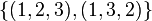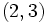# Modular representation theory of symmetric group:S3 at 3

View modular representation theory of particular groups | View other specific information about symmetric group:S3

This article describes the modular representation theory for symmetric group:S3 at the prime three, i.e., for fields of characteristic three, specifically field:F3 and its extensions.

For more information on the (modular) linear representation theory in characteristic two, see modular representation theory of symmetric group:S3 at 2.

For more information on the linear representation theory in other characteristics, see linear representation theory of symmetric group:S3.

## Irreducible representations

There are two irreducible representations, both of them arising from irreducible representations in characteristic zero, namely:

Name of representation type Number of representations of this type Field of realization Kernel Degree Liftable to ordinary representation (characteristic zero)?
trivial 1 field:F3 whole group 1 Yes (lift is also called trivial representation)
sign 1 field:F3 A3 in S3 1 Yes (lift is also called sign representation)

### Trivial representation

The trivial or principal representation is a one-dimensional representation sending every element of the symmetric group to the identity matrix of order one.

### Sign representation

The sign representation or alternating representation sends all the even permutations (the identity element and the two 3-cycles) to the matrix$(1)$ and the three 2-cycles to the matrix$(-1)$. It arises by reducing modulo 3 the sign representation in characteristic zero.

## Character table

The character table (taking values in field:F3) is as follows:

Rep/Conj class$()$ ($3$-regular)$\{ (1,2), (1,3), (2,3) \}$ ($3$-regular)$\{ (1,2,3), (1,3,2) \}$ (not$3$-regular)
trivial 1 1 1
sign 1 -1 1

## Brauer characters

Symmetric group:S3 is a rational representation group, in the sense that all irreducible representations are realized with integer entries. Further, all irreducible representations in characteristic three are realized over field:F3.

Moreover, the relevant eigenvalues do not require adjoining roots of unity, because the fields already come equipped with square roots of unity.

### Brauer character table

There are two 3-regular conjugacy classes: the identity element and the 2-cycles. There are two Brauer characters: arising from the trivial and the sign representation respectively.

Irreducible representation in characteristic three whose Brauer character we are computing Irreducible representation in characteristic zero whose character equals the Brauer character Value of Brauer character on identity element Value of Brauer character on conjugacy class of$(1,2)$,$(1,3)$, and$(2,3)$
trivial representation trivial representation 1 1
sign representation sign representation 1 -1

## GAP implementation

The character degrees can be computed using GAP's CharacterDegrees function as follows:

```gap> CharacterDegrees(SymmetricGroup(3),3);
[ [ 1, 2 ] ]```

The character table can be computed using GAP's CharacterTable function as follows:

```gap> Irr(CharacterTable(SymmetricGroup(3),3));
[ Character( BrauerTable( Sym( [ 1 .. 3 ] ), 3 ), [ 1, -1 ] ),
Character( BrauerTable( Sym( [ 1 .. 3 ] ), 3 ), [ 1, 1 ] ) ]```